# Wind Turbine Control Methods

## Overview

This document explores the fundamental concepts and control methods/techniques for wind turbine control systems. Wind turbine control is necessary to ensure low maintenance costs and efficient performance. The control system also guarantees safe operation, optimizes power output, and ensures long structural life. Turbine rotational speed and the generator speed are two key areas that you must control for power limitation and optimization. The “Control Methods” and “Control Strategies” sections of this document explain which techniques to use and how to manage these areas.

## Key Concepts

Wind Turbine Operation

A wind turbine is a revolving machine that converts the kinetic energy from the wind into mechanical energy. This mechanical energy is then converted into electricity that is sent to a power grid. The turbine components responsible for these energy conversions are the rotor and the generator.

The rotor is the area of the turbine that consists of both the turbine hub and blades. As wind strikes the turbine’s blades, the hub rotates due to aerodynamic forces. This rotation is then sent through the transmission system to decrease the revolutions per minute. The transmission system consists of the main bearing, high-speed shaft, gearbox, and low-speed shaft. The ratio of the gearbox determines the rotation division and the rotation speed that the generator sees. For example, if the ratio of the gearbox is N to 1, then the generator sees the rotor speed divided by N. This rotation is finally sent to the generator for mechanical-to-electrical conversion.

Figure 1 shows the major components of a wind turbine: gearbox, generator, hub, rotor, low-speed shaft, high-speed shaft, and the main bearing.  The purpose of the hub is to connect the blades’ servos that adjust the blade direction to the low-speed shaft. The rotor is the area of the turbine that consists of both the hub and blades. The components are all housed together in a structure called the nacelle.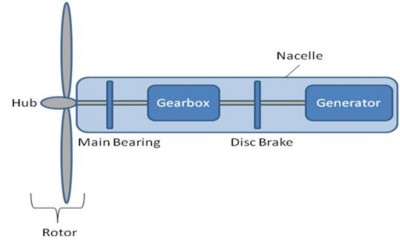Figure 1 . The Major Components of a Wind Turbine

Angle of Attack

The amount of surface area available for the incoming wind is key to increasing aerodynamic forces on the rotor blades. The angle at which the blade is adjusted is referred to as the angle of attack, α. This angle is measured with respect to the incoming wind direction and the chord line of the blade. There is also a critical angle of attack, αcritical, where air no longer streams smoothly over the blade’s upper surface. Figure 2 shows the critical angle of attack with respect to the blade.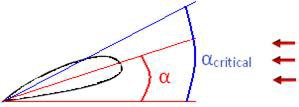Figure 2. The Critical Angle of Attack (αcritical) with Respect to the Blade

Power and Efficiency

This section explains what affects the power extracted from the wind and the efficiency of this process.   Consider Figure 3 as a model of the turbine’s interaction with the wind. This diagram indicates that wind exists on either side of the turbine, and the proper balance between rotational speed and the velocity of wind are critical to regulate performance.  The balance between rotational speed and wind velocity, referred to as the tip speed ratio, is calculated using Equation 1.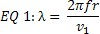Where :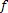is the blades frequency of rotation (Hz)is the length of a blade (m)

Equation 1. Calculating the Tip Speed Ratio

The efficiency of a wind turbine is called the power coefficient, or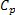. Theoretically, the power coefficient is calculated as the ratio of actual to ideal extracted power. You can find this calculation in Equation 2. Also, you can adjustby controlling the angle of attack, α, and the tip speed ratio,.  The calculation for this case is shown in Equation 3. In Equation 3, c1-c6 and x are coefficients that wind turbine manufacturer should provide. Note that the maximum power coefficient that you can achieve with any turbine is .59, or the Betz limit.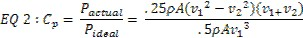Equation 2. The power coefficient is calculated as the ratio of actual to ideal extracted power.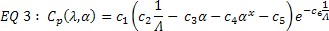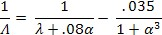Equation 3.You can adjust the  by controlling the angle of attack, α, and the tip speed ratio.

Finally, you can calculate the usable power from the wind using Equation 5. From this equation, you can see that the main drivers for usable power are the blade length and wind speed.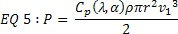Where: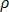= density of air (1.2929 kg/m3)

Equation 5. Calculating Usable Power from the Wind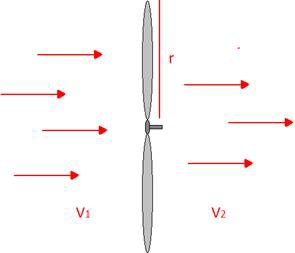Figure 3. Model of the Turbine’s Interaction with the Wind

The Power Curve

It is important to understand the relationship between power and wind speed to determine the required control type, optimization, or limitation. The power curve, a plot you can use for this purpose, specifies how much power you can extract from the incoming wind. Figure 4 contains an ideal wind turbine power curve.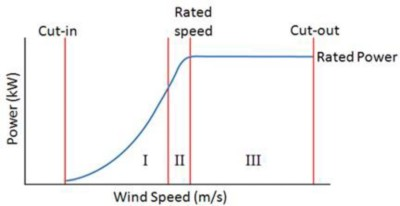Figure 4. Ideal Wind Turbine Power Curve

The cut-in and cut-out speeds are the operating limits of the turbine. By staying in this range, you ensure that the available energy is above the minimum threshold and structural health is maintained. The rated power, a point provided by the manufacturer, takes both energy and cost into consideration. Also, the rated wind speed is chosen because speeds above this point are rare. Typically, you can assume that a turbine design that extracts the bulk of energy above the rated wind speed is not cost-effective.

From Figure 4, you can see that the power curve is split into three distinct regions. Because Region I consists of low wind speeds and is below the rated turbine power, the turbine is run at the maximum efficiency to extract all power. In other words, the turbine controls with optimization in mind. On the other hand, Region III consists of high wind speeds and is at the rated turbine power. The turbine then controls with limitation of the generated power in mind when operating in this region. Finally, Region II is a transition region mainly concerned with keeping rotor torque and noise low.

## Control Methods

You can use different control methods to either optimize or limit power output. You can control a turbine by controlling the generator speed, blade angle adjustment, and rotation of the entire wind turbine. Blade angle adjustment and turbine rotation are also known as pitch and yaw control, respectively. A visual representation of pitch and yaw adjustment is shown in Figures 5 and 6.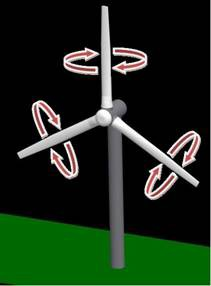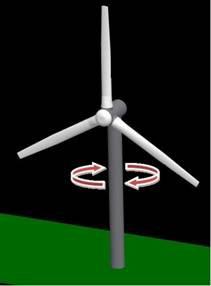The purpose of pitch control is to maintain the optimum blade angle to achieve certain rotor speeds or power output. You can use pitch adjustment to stall and furl, two methods of pitch control. By stalling a wind turbine, you increase the angle of attack, which causes the flat side of the blade to face further into the wind. Furling decreases the angle of attack, causing the edge of the blade to face the oncoming wind. Pitch angle adjustment is the most effective way to limit output power by changing aerodynamic force on the blade at high wind speeds.

Yaw refers to the rotation of the entire wind turbine in the horizontal axis. Yaw control ensures that the turbine is constantly facing into the wind to maximize the effective rotor area and, as a result, power.  Because wind direction can vary quickly, the turbine may misalign with the oncoming wind and cause power output losses. You can approximate these losses with the following equation:

EQ 6: ∆P=α cos(ε)                 Where ∆P is the lost power and ε is the yaw error angle

The final type of control deals with the electrical subsystem. You can achieve this dynamic control with power electronics, or, more specifically, electronic converters that are coupled to the generator. The two types of generator control are stator and rotor. The stator and rotor are the stationary and nonstationary parts of a generator, respectively. In each case, you disconnect the stator or rotor from the grid to change the synchronous speed of the generator independently of the voltage or frequency of the grid. Controlling the synchronous generator speed is the most effective way to optimize maximum power output at low wind speeds.

Figure 7 shows a system-level layout of a wind energy conversion system and the signals used. Notice that control is most effective by adjusting pitch angle and controlling the synchronous speed of the generator.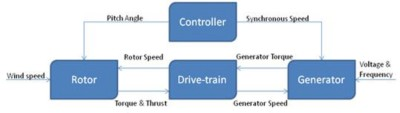Figure 7. System-Level Layout of a Wind Energy System

## Control Strategies

Recall that controlling the pitch of the blade and speed of the generator are the most effective methods to adjust output power. The following control strategies use pitch and generator speed control to manage turbine functionality throughout the power curve: fixed-speed fixed-pitch, fixed-speed variable-pitch, variable-speed fixed-pitch, and variable-speed variable-pitch. Figure 8 shows the power curves for different control strategies explained below, with variable-speed variable-pitch, VS-VP, being the ideal curve.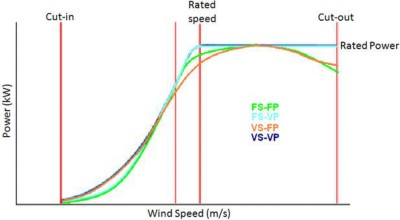Figure 8. Power Curves for Different Control Strategies (Variable-speed variable-pitch, VS-VP, is the ideal curve.)

Fixed-speed fixed-pitch (FS-FP) is the one configuration where it is impossible to improve performance with active control. In this design, the turbine’s generator is directly coupled to the power grid, causing the generator speed to lock to the power line frequency and fix the rotational speed. These turbines are regulated using passive stall methods at high wind speeds. The gearbox ratio selection becomes important for this passive control because it ensures that the rated power is not exceeded. Figure 8 shows the power curve for FS-FP operation.

From the figure, it is apparent that the actual power does not match the ideal power, implying that there is lower energy capture. Notice that the turbine operates at maximum efficiency only at one wind speed in the low-speed region. The rated power of the turbine is achieved only at one wind speed as well. This implies poor power regulation as a result of constrained operations.

Fixed-speed variable-pitch (FS-VP) configuration operates at a fixed pitch angle below the rated wind speed and continuously adjusts the angle above the rated wind speed. To clarify, fixed-speed operation implies a maximum output power at one wind speed. You can use both feather and stall pitch control methods in this configuration to limit power. Keep in mind that feathering takes a significant amount of control design and stalling increases unwanted thrust force as stall increases. Figure 8 shows the power curve for FS-VP using either feather or stall control.

Below the rated wind speed, the FS-VP turbine has a near optimum efficiency around Region II.  Exceeding the rated wind speed, the pitch angles are continuously changed, providing little to no loss in power.

Variable-speed fixed-pitch (VS-FP) configuration continuously adjusts the rotor speed relative to the wind speed through power electronics controlling the synchronous speed of the generator. This type of control assumes that the generator is from the grid so that the generator’s rotor and drive-train are free to rotate independently of grid frequency. Fixed-pitch relies heavily on the blade design to limit power through passive stalling. Figure 8 shows the power curve for VS-FP.

Figure 8 shows that power efficiency is maximized at low wind speeds, and you can achieve rated turbine power only at one wind speed. Passive stall regulation plays a major role in not achieving the rated power and can be attributed to poor power regulation above the rated wind speed. In lower wind speed cases, VS-FP can capture more energy and improve power quality.

Variable-speed variable-pitch (VS-VP) configuration is a derivation of VS-FP and FS-VP. Operating below the rated wind speed, variable speed and fixed pitch are used to maximize energy capture and increase power quality. Operating above the rated wind speed, fixed speed and variable pitch permit efficient power regulation at the rated power. VS-VP is the only control strategy that theoretically achieves the ideal power curve shown in Figure 8.

## Summary

This document covered some essential wind energy concepts, such as the angle of attack and the power coefficient, as well as different control methods and strategies.  Pitch, Yaw, and Rotational Speed Control were the main control methods used to optimize or limit the power extracted from the wind.   Wind turbine control is essential for optimal performance, safe operation, and structural stability.